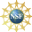# 8. Properties of Curves

## 3. Speed

The arc length function allows us to give an interpretation to the length of the velocity vector. By definition, the speed is the rate of change of the distance along the curve, i.e. the rate of change of the arc length parameter: $speed=\dfrac{ds}{dt}=\dfrac{d}{dt}\int_a^t |\vec{v}|\,dt$ By the fundamental theorem of calculus, the derivative of an integral with respect to the upper limit is the integrand. Thus: $speed=\dfrac{ds}{dt}=|\vec{v}|$ In other words, the length of the velocity vector is the speed. You probably heard it many times before that speed is the magnitude of the velocity, but this proves it.

Previously we found the velocity vector for the Circle, $$\vec{r}(\theta)=(4\cos\theta,4\sin\theta,2)$$, is: $\vec{v}(\theta)=\dfrac{d\vec{r}}{d\theta} =\langle -4\sin\theta,4\cos\theta,0\rangle$ Find the speed of the curve.

$$\dfrac{ds}{dt}=|\vec{v}|=4$$

The speed is: \begin{aligned} \dfrac{ds}{dt} &=|\vec{v}| =\sqrt{(-4\sin\theta)^2+(4\cos\theta)^2} \\ &=\sqrt{16\sin^2\theta+16\cos^2\theta} =\sqrt{16} =4 \end{aligned}

Previously we found the velocity vector for the Helix, $$\vec{r}(\theta)=(4\cos\theta,4\sin\theta,3\theta)$$, is: $\vec{v}(\theta)=\dfrac{d\vec{r}}{d\theta} =\langle -4\sin\theta,4\cos\theta,3\rangle$ Find the speed of the curve.

$$\dfrac{ds}{dt}=|\vec{v}|=5$$

The speed is: \begin{aligned} \dfrac{ds}{dt} &=|\vec{v}| =\sqrt{((-4\sin\theta)^2+(4\cos\theta)^2+9} \\ &=\sqrt{16\sin^2\theta+16\cos^2\theta+9} =\sqrt{16+9}=5 \end{aligned}

Previously we found the velocity vector for the Twisted Cubic, $$\vec{r}(t)=\left(t,t^2,\dfrac{2}{3}t^3\right)$$, is: $\vec{v}(t)=\dfrac{d\vec{r}}{dt}=\langle 1,2t,2t^2\rangle$ Find the speed of the curve.

The quantity inside the square root is a perfect square.
This is, in fact, the reason the coefficient $$\dfrac{2}{3}$$ is included in the formula for the standard twisted cubic.

$$\dfrac{ds}{dt}=|\vec{v}|=2t^2+1$$

The speed is: \begin{aligned} \dfrac{ds}{dt} &=|\vec{v}| =\sqrt{1^2+(2t)^2+(2t^2)^2} \\ &=\sqrt{1+4t^2+4t^{4}} =\sqrt{(2t^2+1)^2} =2t^2+1 \end{aligned}

On the previous page, we mentioned that it may be difficult to reparametrize a curve in terms of the arc length parameter, $$s$$. However, it is usually easy to differentiate with respect to the arc length parameter, $$s$$, provided you can differentiate with respect to the original parameter $$t$$. In particular, if $$f(t)$$ is a function of $$t$$ for which you can compute the derivative $$\dfrac{df}{dt}$$, then by the chain rule: $\dfrac{df}{ds}=\dfrac{df}{dt}\dfrac{dt}{ds}=\dfrac{1}{ds/dt} \dfrac{df}{dt}=\dfrac{1}{|\vec{v}|}\dfrac{df}{dt}$ Conversely, $\dfrac{df}{dt}=\dfrac{df}{ds}\dfrac{ds}{dt}=|\vec{v}|\dfrac{df}{ds}$ These two relations will be very useful later in this chapter.

Supported in part by NSF Grant #1123255